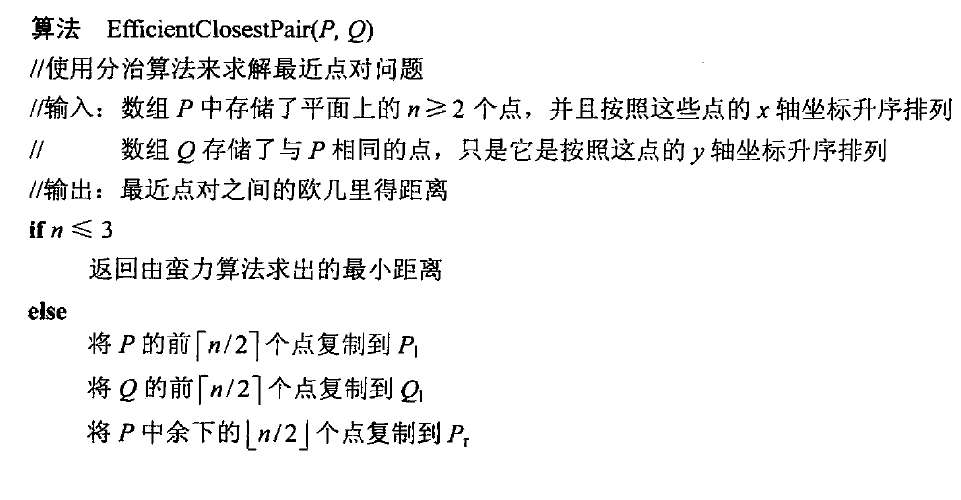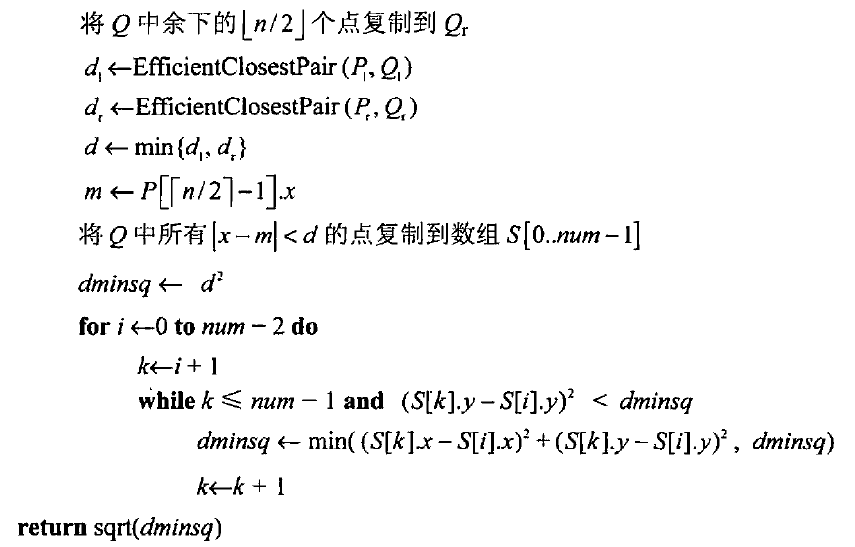# 算法笔记-分治法

Posted by MaggicQ on May 29, 2017

# 分治法

## 主定理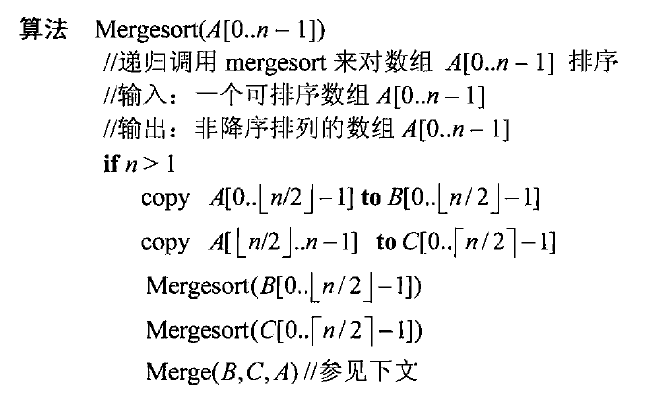## 合并排序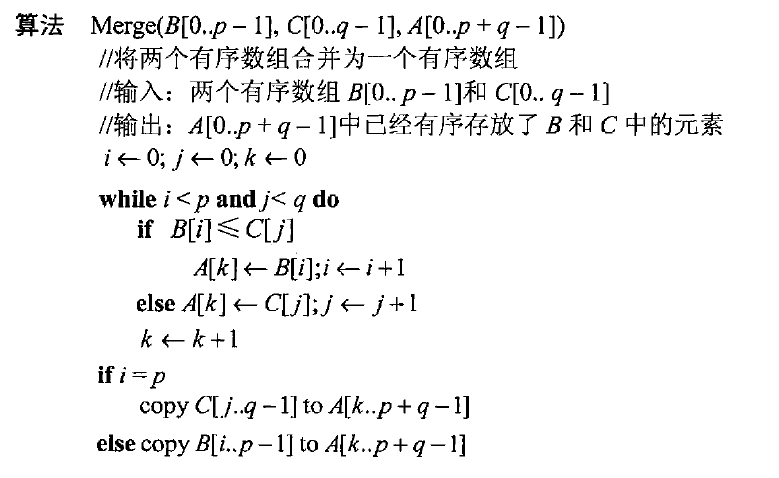$C_{worst}(n) = nlog_2n - n + 1$

### 快速排序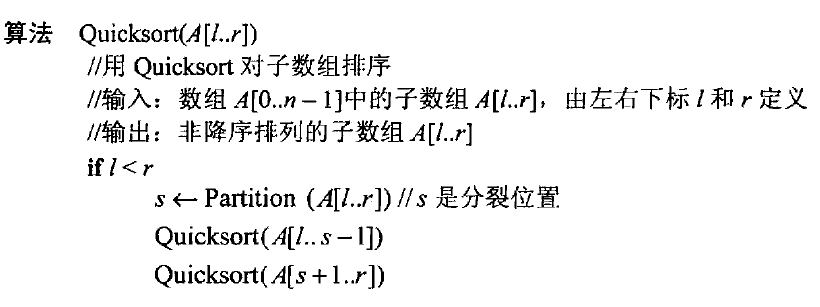$C_{worst}(n) \in \Theta(n^2)$

### 二叉树遍历及其相关特性

$T_L$ 和 $T_R$ 的不相交二叉树构成，这两科二叉树分别为根的左右子树。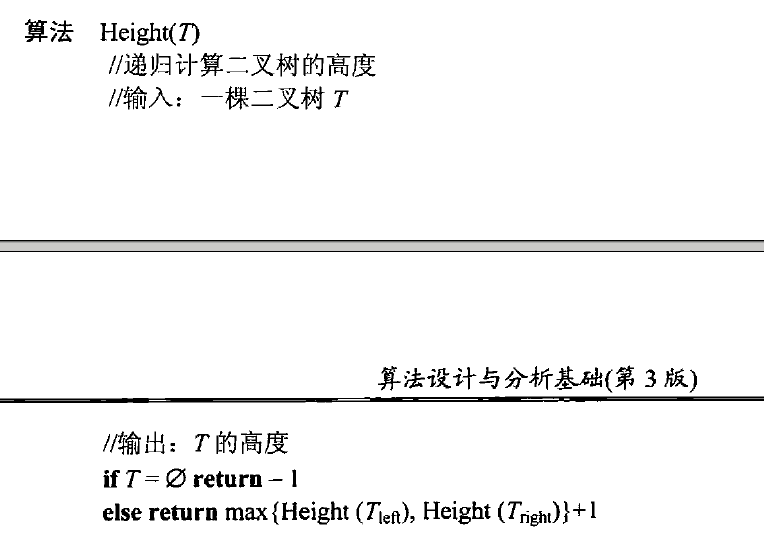## 大整数乘法和 Strassen矩阵乘法

### 大整数乘法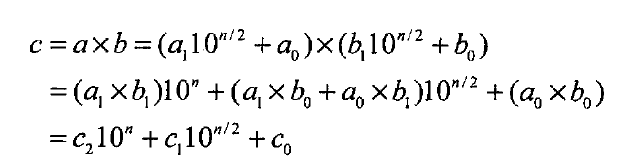$c_0 = a_0 \times b_0$

$c_1 = (a_1 + a_0) \times (b_1 + b_0) - (c_2 + c_0)$

$n> 1 时， M(n) = 3M(n/2), M(1) = 1$

### Strassen矩阵乘法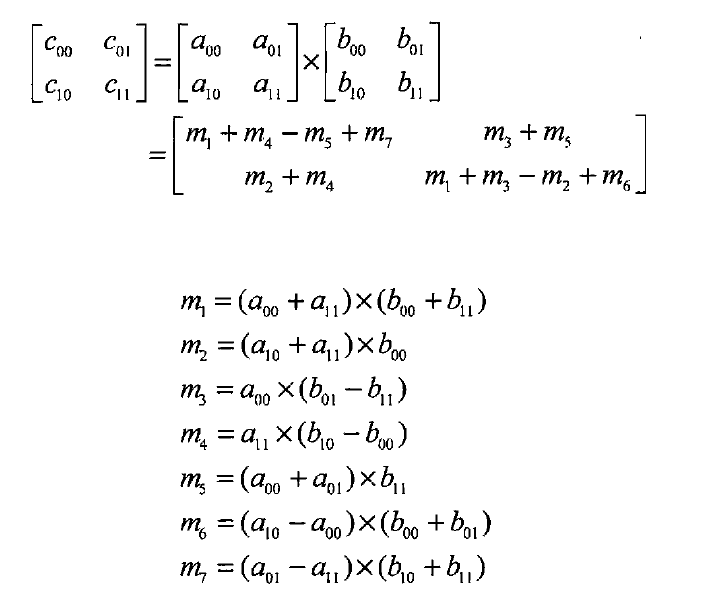$n > 1, M(n) = 7M(n/2) , M(1) =1$

## 用分治法解最近对问题和凸包问题

### 解决最近对问题

$2 \leq n \leq 3$: 使用 蛮力算法进行求解

$n > 3$ : 可以利用点集在 $x$ 轴方向上的中位数 $m$ ,在该处